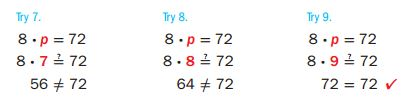Homework Explained - Math Practice 101Dear guest, you are not a registered member. As a guest, you only have read-only access to our books, tests and other practice materials.

As a registered member you can:

Registration is free and doesn't require any type of payment information. Click here to Register.
Go to page:
Chapter 7:Equations;Lesson 1: Equations

Example

Mason bought 72 sticks of gum. There are 8 stlcks of gum in each package. Use the guess, check and revise strategy to solve the equation 8 x p = 72 to find p, the number of packages Mason bought.
Use the  guess, check, and revise strategy.So, Mason bought 9 packages of gum

Identify the solution of each equation from the list given.

• Question 1

9 + w = 17;

7, 8, 9

• Question 2

8 ÷ c = 8;

0,1,2

Solve each equation mentally.

• Question 3

x — 11 = 23

• Question 5

Mississippi and Georgia have a total of 21 electoral votes. Mississippi has 6 electoral votes. Use mental math or the guess, check, and revise strategy to solve the equation 6 + g = 21 to find g, the number of electoral votes Georgia has.

• Question 6

Riley and her sister collect stickers. Riley has 220 stickers in her sticker collection. Her sister has 55 stickers in her collection. Riley has how many times as many stickers as her sister? Use mental math or the guess, check and revise strategy to solve the equation 55x = 220.

• Question 7

Building on the Essential Question How do you solve an equation?

Yes, email page to my online tutor. (if you didn't add a tutor yet, you can add one here)